# Algebra Bricks (1)

In this worksheet, students add and subtract expressions to find the missing Algebra bricks.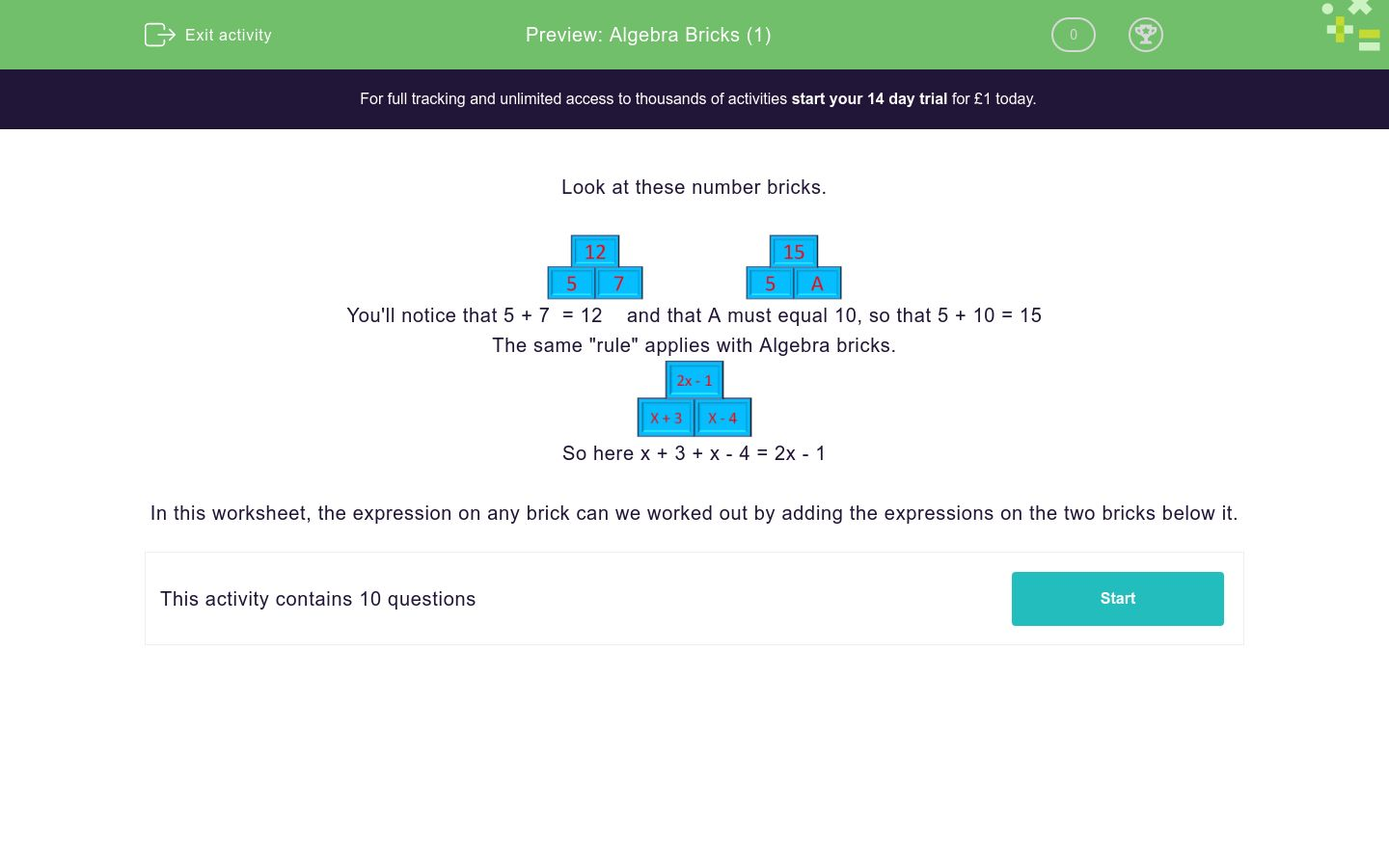Key stage:  KS 3

Curriculum topic:   Algebra

Curriculum subtopic:   Understand Expressions, Equations, Inequalities, Terms and Factors

Difficulty level:### QUESTION 1 of 10

Look at these number bricks.You'll notice that 5 + 7  = 12    and that A must equal 10, so that 5 + 10 = 15

The same "rule" applies with Algebra bricks.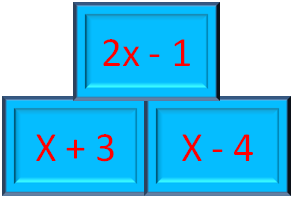So here x + 3 + x - 4 = 2x - 1

In this worksheet, the expression on any brick can we worked out by adding the expressions on the two bricks below it.

Work out the value of A in these Algebra bricks:x - 2

2x - 8

2x - 2

Work out the value of A in these Algebra bricks:2x - 1

2x - 9

4x - 1

Work out the value of A in these Algebra bricks:x + 13

x + 5

3x + 13

Work out the value of A in these Algebra bricks: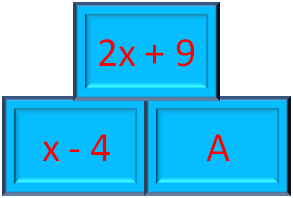x + 13

x + 5

3x + 5

Work out the value of A in these Algebra bricks: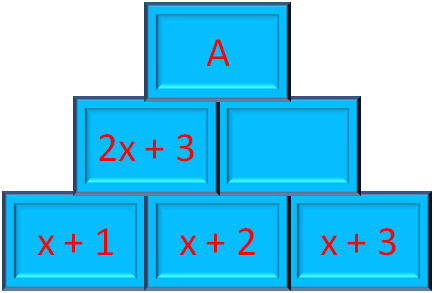4x + 13

4x + 8

3x + 5

Work out the value of A in these Algebra bricks:8x + 13

4x + 8

8x + 8

Work out the value of A in these Algebra bricks:8x + 13

8x + 8

8x + 6

Work out the value of A in these Algebra bricks: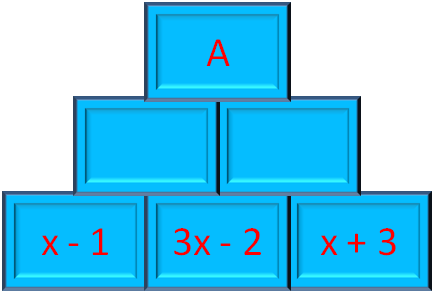8x - 2

8x + 8

8x + 7

Work out the value of A in these Algebra bricks: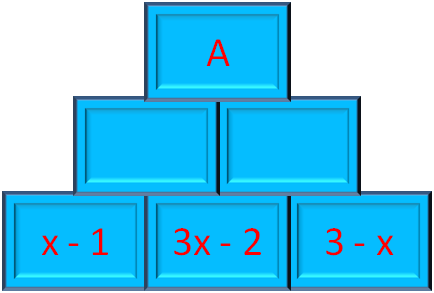8x - 2

6x - 2

6x + 7

Work out the value of A in these Algebra bricks:4x

6x - 2

4x + 7

• Question 1

Work out the value of A in these Algebra bricks:2x - 2
• Question 2

Work out the value of A in these Algebra bricks:4x - 1
• Question 3

Work out the value of A in these Algebra bricks:x + 5
• Question 4

Work out the value of A in these Algebra bricks:x + 13
• Question 5

Work out the value of A in these Algebra bricks:4x + 8
• Question 6

Work out the value of A in these Algebra bricks:8x + 8
• Question 7

Work out the value of A in these Algebra bricks:8x + 6
• Question 8

Work out the value of A in these Algebra bricks:8x - 2
• Question 9

Work out the value of A in these Algebra bricks:6x - 2
• Question 10

Work out the value of A in these Algebra bricks:4x
---- OR ----

Sign up for a £1 trial so you can track and measure your child's progress on this activity.

### What is EdPlace?

We're your National Curriculum aligned online education content provider helping each child succeed in English, maths and science from year 1 to GCSE. With an EdPlace account you’ll be able to track and measure progress, helping each child achieve their best. We build confidence and attainment by personalising each child’s learning at a level that suits them.

Get started